# Average

What is the average speed of the car, where half of the distance covered passed at speed 66 km/h and the other half at 86 km/h.

v =  74.68 km/h

### Step-by-step explanation: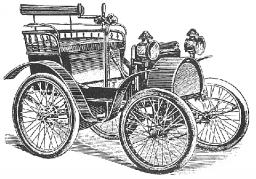Did you find an error or inaccuracy? Feel free to write us. Thank you!Tips to related online calculators
Looking for help with calculating arithmetic mean?
Looking for calculator of harmonic mean?
Looking for a statistical calculator?
Do you want to convert velocity (speed) units?

#### You need to know the following knowledge to solve this word math problem:

We encourage you to watch this tutorial video on this math problem:

## Related math problems and questions:

• Average speed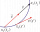The car drove on one section of the highway for half an hour at a speed of 80 km/h. Then he reduced his speed to 60km/h. It went three-quarters of an hour at a speed of 60 km/h. What was the average speed of the car?
• Two citiesThe car goes from city A to city B at an average speed of 70 km/h, back at an average speed of 50 km/h. If it goes to B and back at an average speed of 60 km/h, the whole ride will take 8 minutes less. What is the distance between cities A and B?
• Average speedThe car drove from city A to city B at a 40 km/h and back to city A at a speed of 80 km/h. What was the average speed of the car?
• Cars motion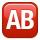From point A car start at the speed of 90 km/h. Against it departs from the point B at the same time another car at 60 km/h. For how long and where they met if the distance between A and B is 180 km?
• Average speedThe truck drove 1/2 of the way on the highway at 80km/h. The other half of the way 20km/h. Calculate the average speed
• Motorcyclist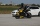From Trutnov, the motorcyclist started at an average speed of 60km/hour. At 12.30hrs the passenger car was started at a speed of 80km/hour. How many hours and at what distance from Trutnov will car catch a motorcycle?
• Car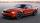The car goes from point A to point B at speed 86 km/h and back 53 km/h. If it goes there and back at speed 67 km/h trip would take 10 minutes shorter. What is the distance between points A and B?
• Forth and back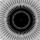Car drive from point A to point B at speed 78 km/h and back at 82 km/h. If went there and back at speed 81 km/h journey would take five minutes less. What is the distance between points A and B?
• Znojmo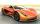From Znojmo to Brno started the truck with a trailer at an average speed 53 km per hour. Against him, 14 minutes later from Brno started the car with an average speed of 1.2-times greater than the truck. How long and how far from Znojmo, they meet if the
• MotionFrom two different locations distant 232 km started against car and bus. The car started at 5:20 with average speed 64 km/h. Bus started at 7:30 with average speed 80 km/h. When they meet? How many kilometers went the bus?
• Cars 2Opava is 360 km distance from Prešov. At 8:00 hour, two cars started against each other from this cities at average speeds 88 km/h and 121 km/h. What hour will it meet and what distance it's travel if the car started from Prešov is faster?
• Where and when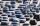The truck left Kremnica at 11:00 h at a speed of 60km/h. At 12:30 h, the passenger car started at an average speed of 80km/h. How many kilometers from Kremnica will the passenger car reach the truck, and when?
• Motion 2At 10 o'clock started from place A car speed at 90 km/h. Twenty minutes after starting another car from place B at a speed 60 km/h . Distance places A and B is 120 kilometers. What time and where do they meet?
• Two cars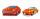From the town A to town B started two cars. The first at 7:00 at average speed 60 km per hour, the second at 10:00 at average speed 100 km per hour. The first car will not stay in B, and on the way back meet the second car at half way from A to B. At what
• Athletic competitionIn a 400 meter athletic competition, a participant covers the distance as given below. find the average speed? first 80 meters 10 m/s next 240 meters 7.5 m/s last 80 meters 10 m/s
• Express train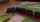International express train drove from Kosice to Teplice. In the first 279 km, the track was repaired, and therefore it was moving at a speed of 10km/h less than it was scheduled to drive. The rest of the 465 km trip has increased the speed by 8 km/h than
• CarThe driver of a car is to get to 608 km distant city. From atlas found that 162 km will have to pass through the cities at an average speed 48 km/h. The remainder of the journey passes outside the cities at average speed 116 km/h. Calculate how many hours